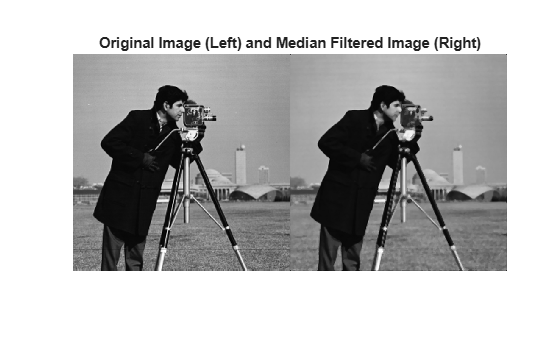# nlfilter

General sliding-neighborhood operations

## Syntax

``B = nlfilter(A,[m n],fun)``
``B = nlfilter(A,'indexed',___)``

## Description

example

````B = nlfilter(A,[m n],fun)` applies the function `fun` to each `m`-by-`n` sliding block of the grayscale image `A`.```
````B = nlfilter(A,'indexed',___)` processes `A` as an indexed image, padding with `0`s if the class of `A` is `uint8`, `uint16`, or `logical`, and padding with `1`s otherwise. Note`nlfilter` can take a long time to process large images. In some cases, the `colfilt` function can perform the same operation much faster. ```

## Examples

collapse all

This example shows how to apply a median filter to an image using `nlfilter`. This example produces the same result as calling `medfilt2` with a 3-by-3 neighborhood.

Read an image into the workspace.

`A = imread('cameraman.tif'); `

Convert the image to `double`.

`A = im2double(A);`

Create the function you want to apply to the image—a median filter.

`fun = @(x) median(x(:));`

Apply the filter to the image.

`B = nlfilter(A,[3 3],fun); `

Display the original image and the filtered image, side-by-side.

```montage({A,B}) title('Original Image (Left) and Median Filtered Image (Right)')```## Input Arguments

collapse all

Image to be filtered, specified as a numeric array of any class supported by `fun`. When `A` is grayscale, it can be any numeric type or `logical`. When `A` is indexed, it can be `logical`, `uint8`, `uint16`, `single`, or `double`.

Data Types: `single` | `double` | `int8` | `int16` | `int32` | `int64` | `uint8` | `uint16` | `uint32` | `uint64` | `logical`

Block size, specified as a 2-element vector of positive integers. `m` is the number of rows and `n` is the number of columns in the block.

Example: `B = nlfilter(A,[3 3],fun);`

Data Types: `single` | `double` | `logical`

Function handle specified as a handle. The function must accept an `m`-by-`n` matrix as input and return a scalar result.

`c = fun(x)`

`c` is the output value for the center pixel in the `m`-by-`n` block `x`. `nlfilter` calls `fun` for each pixel in `A`. `nlfilter` zero-pads the `m`-by-`n` block at the edges, if necessary.

Data Types: `function_handle`

## Output Arguments

collapse all

Filtered image, returned as numeric array. The class of `B` depends on the class of the output from `fun`.